# TPACK Model LESSON PLAN IN MATHEMATICS 10 I

• Slides: 22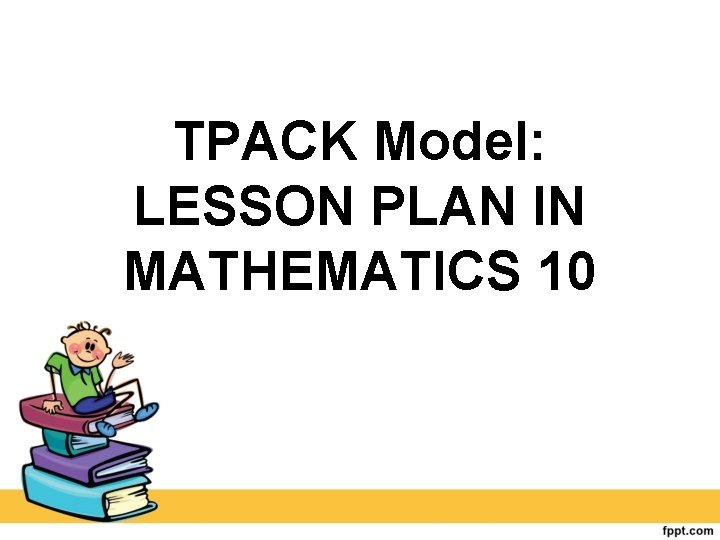TPACK Model: LESSON PLAN IN MATHEMATICS 10I. OBJECTIVES Content Standard: a. Demonstrates understanding of key concepts of circle and coordinate geometry. Performance Standard: a. Is able to formulate and find solutions to challenging situations involving circles and other related terms in different disciplines through appropriate and accurate representations.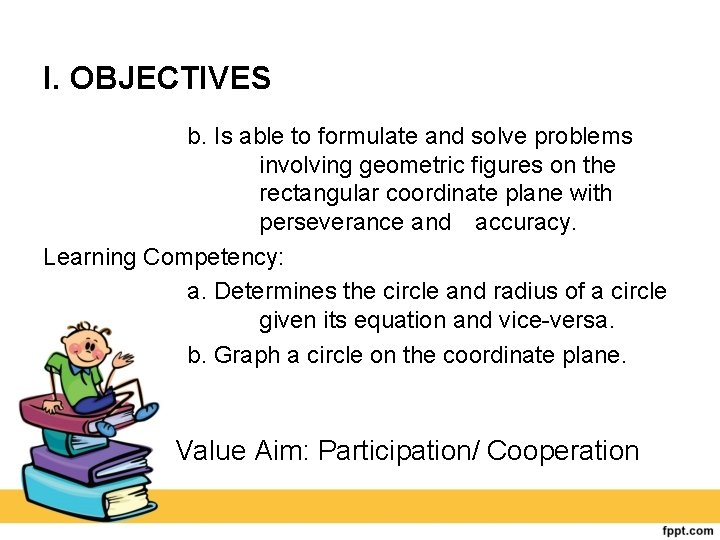I. OBJECTIVES b. Is able to formulate and solve problems involving geometric figures on the rectangular coordinate plane with perseverance and accuracy. Learning Competency: a. Determines the circle and radius of a circle given its equation and vice versa. b. Graph a circle on the coordinate plane. Value Aim: Participation/ Cooperation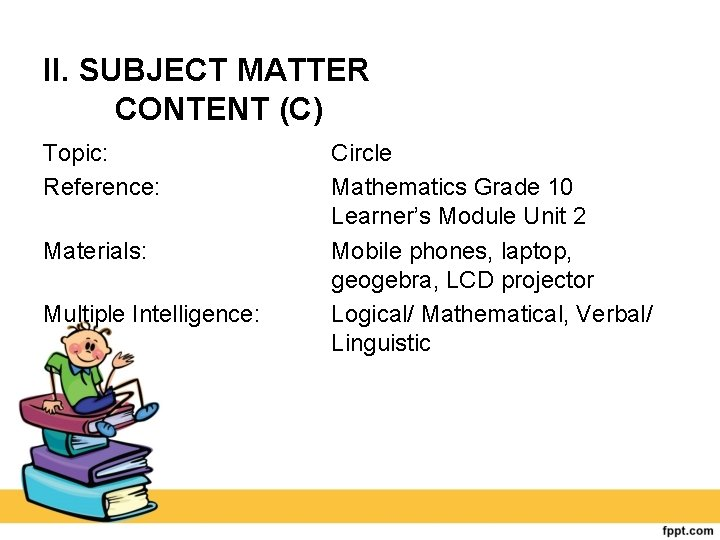II. SUBJECT MATTER CONTENT (C) Topic: Reference: Materials: Multiple Intelligence: Circle Mathematics Grade 10 Learner’s Module Unit 2 Mobile phones, laptop, geogebra, LCD projector Logical/ Mathematical, Verbal/ LinguisticIII. PROCEDURE Lesson Proper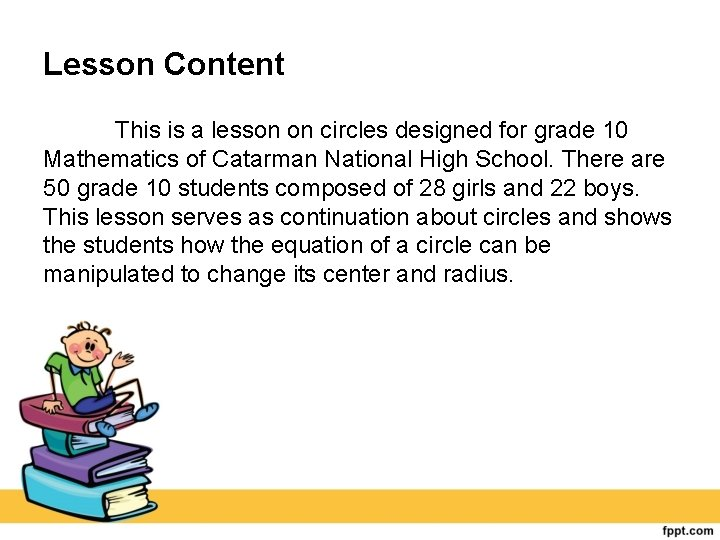Lesson Content This is a lesson on circles designed for grade 10 Mathematics of Catarman National High School. There are 50 grade 10 students composed of 28 girls and 22 boys. This lesson serves as continuation about circles and shows the students how the equation of a circle can be manipulated to change its center and radius.Lesson Content •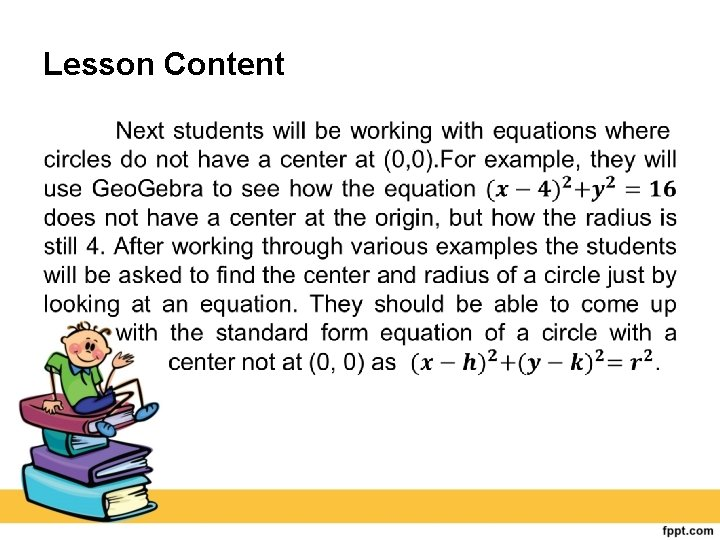Lesson Content •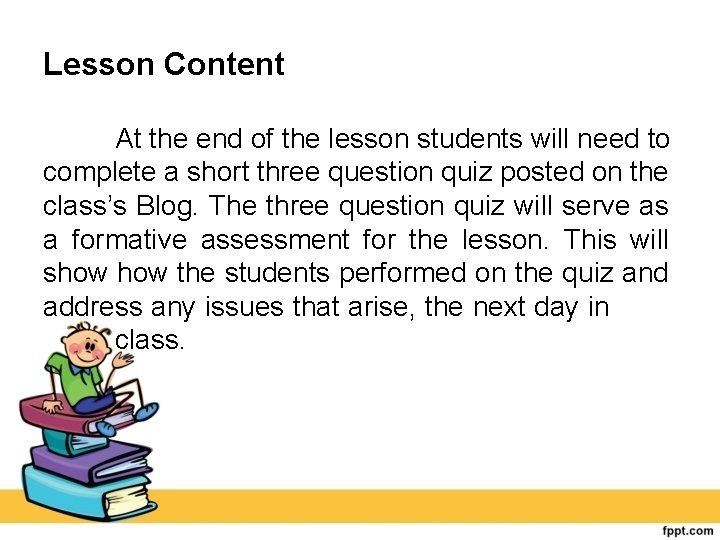Lesson Content At the end of the lesson students will need to complete a short three question quiz posted on the class’s Blog. The three question quiz will serve as a formative assessment for the lesson. This will show the students performed on the quiz and address any issues that arise, the next day in class.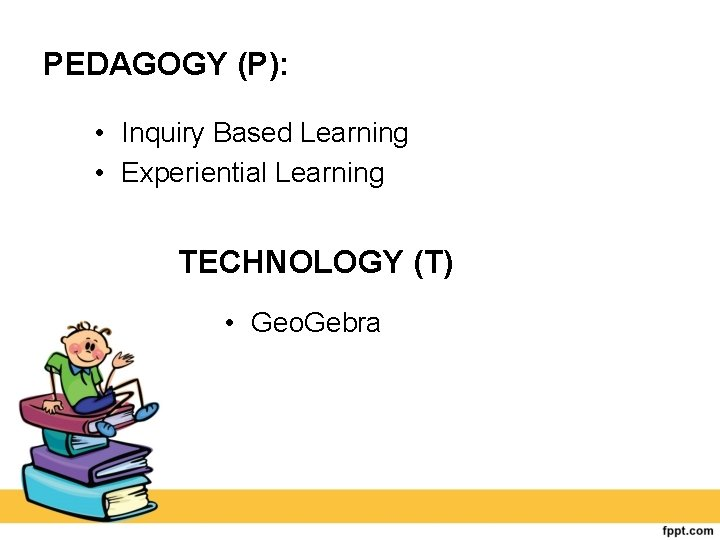PEDAGOGY (P): • Inquiry Based Learning • Experiential Learning TECHNOLOGY (T) • Geo. GebraPEDAGOGY (P): • Inquiry Based Learning • Experiential Learning TECHNOLOGY (T) • Geo. Gebra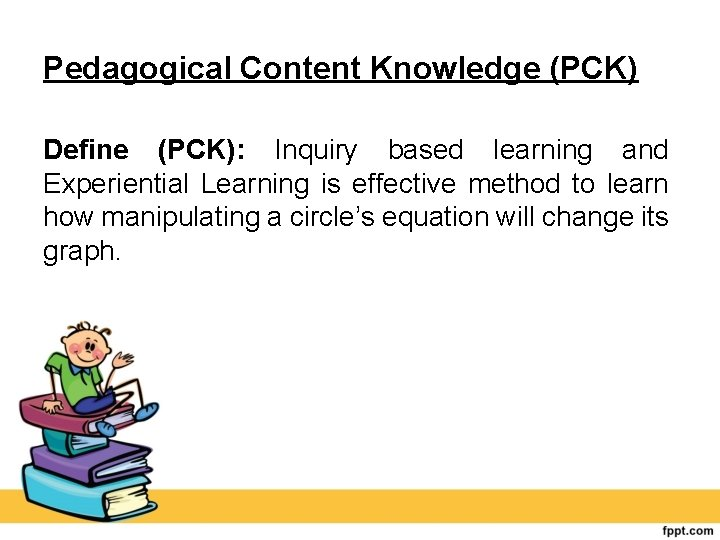Pedagogical Content Knowledge (PCK) Define (PCK): Inquiry based learning and Experiential Learning is effective method to learn how manipulating a circle’s equation will change its graph.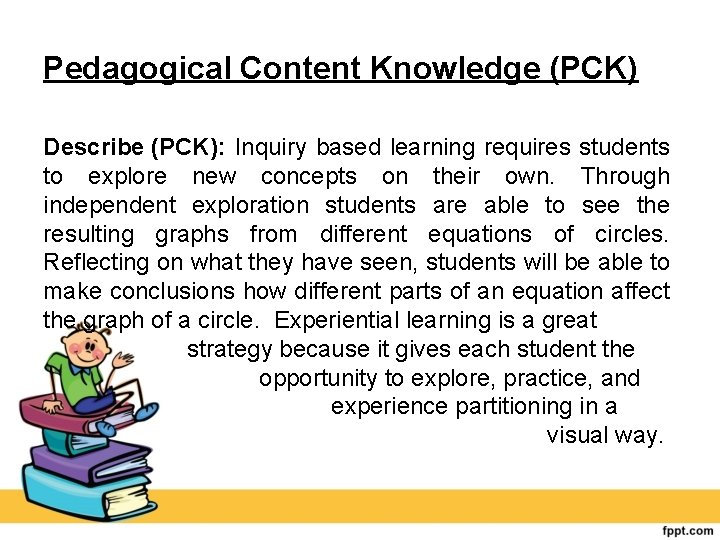Pedagogical Content Knowledge (PCK) Describe (PCK): Inquiry based learning requires students to explore new concepts on their own. Through independent exploration students are able to see the resulting graphs from different equations of circles. Reflecting on what they have seen, students will be able to make conclusions how different parts of an equation affect the graph of a circle. Experiential learning is a great strategy because it gives each student the opportunity to explore, practice, and experience partitioning in a visual way.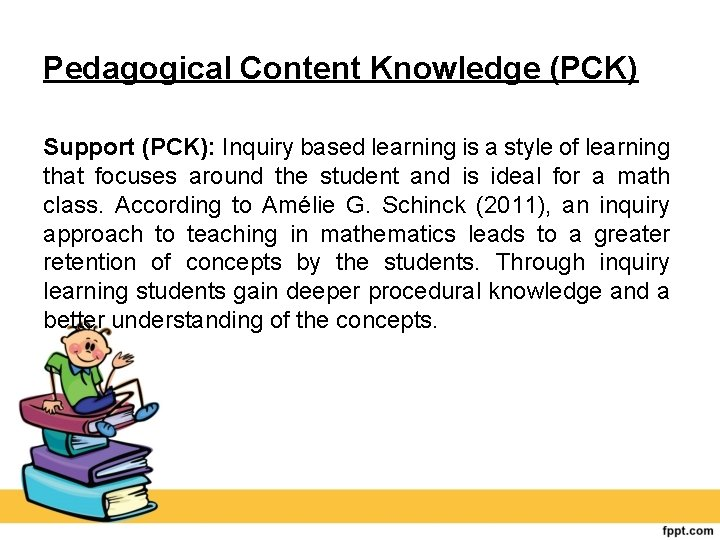Pedagogical Content Knowledge (PCK) Support (PCK): Inquiry based learning is a style of learning that focuses around the student and is ideal for a math class. According to Amélie G. Schinck (2011), an inquiry approach to teaching in mathematics leads to a greater retention of concepts by the students. Through inquiry learning students gain deeper procedural knowledge and a better understanding of the concepts.Pedagogical Content Knowledge (PCK) Hartshorn and Boren state that “research suggests that manipulative are particularly useful in helping children move from the concrete to the abstract level. Teachers must choose activities and manipulative carefully to support the introduction of abstract symbols” (1990).Technological Content Knowledge (TCK): Define (TCK): Geo. Gebra is a technology that supports the content goals for this lesson. Describe (TCK): Geo. Gebra is a technology that mixes geometry with algebra. For this lesson students will be working with concepts from both geometry and algebra. They will be working with circles and seeing how equations can change the graph of a circle. Since this lesson incorporates a combination of geometry and algebra, Geo. Gebra is the ideal technology to use.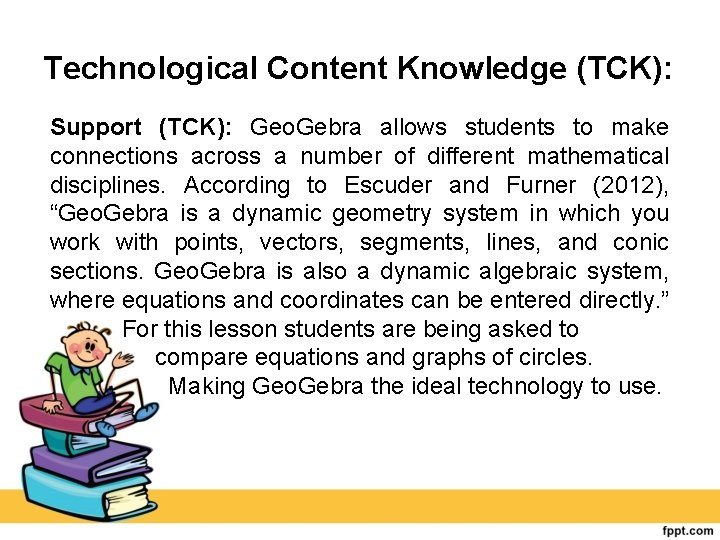Technological Content Knowledge (TCK): Support (TCK): Geo. Gebra allows students to make connections across a number of different mathematical disciplines. According to Escuder and Furner (2012), “Geo. Gebra is a dynamic geometry system in which you work with points, vectors, segments, lines, and conic sections. Geo. Gebra is also a dynamic algebraic system, where equations and coordinates can be entered directly. ” For this lesson students are being asked to compare equations and graphs of circles. Making Geo. Gebra the ideal technology to use.Technological Pedagogical Content Knowledge (TPK) Define (TPK): Geo. Gebra is an effective technology tool to facilitate inquiry based learning. Describe (TPK): Geo. Gebra allows students to type in equations for various circles and see the resulting graphs. This technology allows students to explore how manipulating equations in various ways affect the resulting graph.Technological Pedagogical Content Knowledge (TPK) Support (TPK): Geo. Gebra technology will allow students to analyze the manipulation of equations more efficiently. According to Milan Sherman (2010), “When technology is used as an amplifier , it performs more efficiently tedious processes that might be done by hand, like computation or the generation of standard representations. In this use of technology, what students do or think about isn’t changed, but can be done with significantly less time and effort. ” The time that Geo. Gebra provides the students will allow them to focus on making conclusions about how the equations and graphs of circles are related.Technological Pedagogical Content Knowledge (TPACK) Define (TPACK): Teaching circles through inquiry based learning and experiential learning using Geo. Gebra creates an environment conducive to learning. Describe (TPACK): They have a basic understanding of circles from their geometry classes, but now they will see how equations can impact the graph of a circle. Using Geo. Gebra students will be able to progress through an inquiry based lesson where they are able to make conclusions about how different components of the equation for a circle impact the resulting graph.Technological Pedagogical Content Knowledge (TPACK) Support (TPACK): In a study performed by Praveen Shadaan and Leong Kwan Eu (2013), it was found that students who were taught circles using Geo. Gebra had an increase in conceptual understanding compared to those not taught with Geo. Gebra. These observations support the decision to teach the students’ circles through inquiry based learning and experiential learning using Geo. Gebra.Prepared by: Shierna R. Gulariza Samuel M. Tenedero Daisy P. Domingo